# Particles

## Density

The density of a substance is defined as its mass per unit volume. If two objects are the same size but different densities, the more dense object will be heavier.

Density can be calculated using the following equation (you DO need to remember this equation for your exam):

# ρ = m ÷ v

ρ = density (kg/m3)

m = mass (kg)

v = volume (m3)

Worked Example

A wooden post has a volume of 0.025m3 and a mass of 20kg. Calculate its density.

ρ = m ÷ v

ρ = 20 ÷ 0.025

ρ = 800kg/m3

Density can have units of kg/m3 or g/cm3

Converting units:

1. 1 kg = 1000g
2. 1m = 100cm
3. 1m3 = 1,000,000 cm3
4. 1000 kg/m3 = 1,000,000g ÷ 1,000,000cm3 = 1g/cm3

## States of Matter

There are 3 states of matter:

1. Solid
2. Liquid
3. Gas

They are defined by the arrangement and movement of their particles.

Solids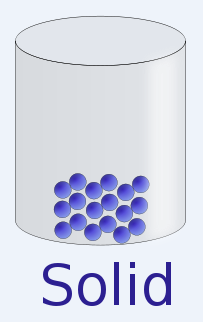1. Particles tightly packed together
2. Vibrate in fixed positions
3. Fixed shape
4. Fixed volume (can not be compressed)
5. High density

Liquids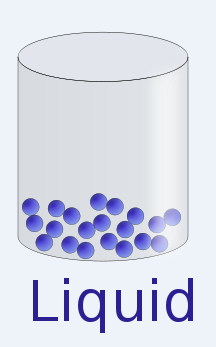1. Particles close together
2. Move around randomly
3. No fixed shape
4. Fixed volume (can not be compressed)

Gases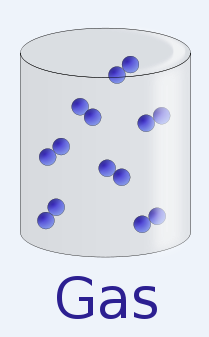1. Particles move around randomly at high speeds
2. Particles far apart
3. No fixed shape
4. No fixed volume (can be compressed)
5. Low density

## Changes of State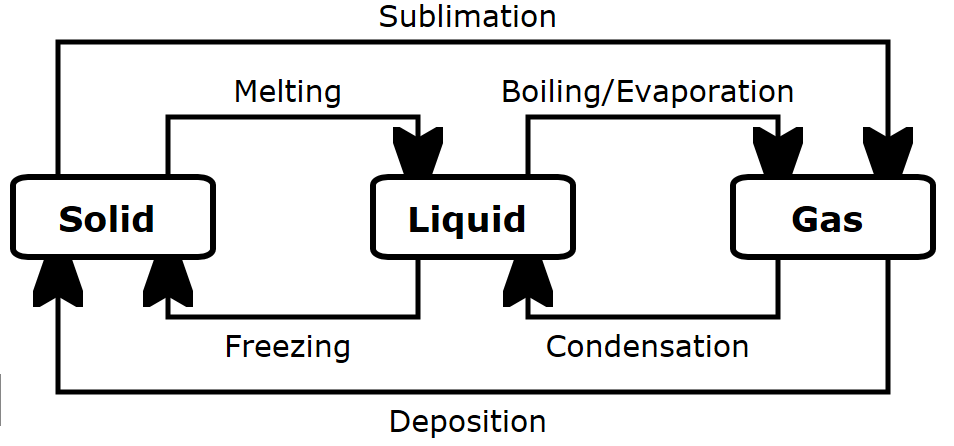When a substance changes state, energy is transferred to or from the substance. Thermal energy is transferred to a solid to cause it to melt. Thermal energy is transferred from a liquid, causing it to freeze.

When a substance changes state, the number of particles remains the same. Therefore the mass of the substance remains the same. This is known as conservation of mass.

As a substance is heated, it’s particles gain kinetic energy allowing them to break free of their positions and melt or evaporate/boil. As a substance cools, it’s particles lose kinetic energy.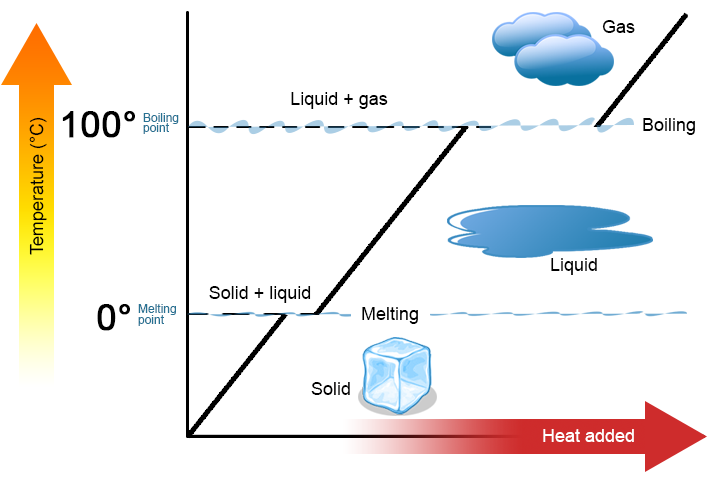As a substance changes state, it’s temperature remains constant even though thermal energy is being transferred to it. This energy is called latent heat.

## Internal Energy

The energy stored in the particles of a substance is called internal energy. Increasing the temperature of a substance increases it’s internal energy.

The internal energy depends on:

1. The kinetic energy of each particle
2. The potential energy of the particles based on the distance between them
3. Video 1
4. Video 2

Internal Energy in solids, liquids and gases:

Solids

1. Strong forces of attraction between particles
2. Particles vibrate in fixed positions
3. When heated, particles vibrate with more kinetic energy
4. If heated enough, the solid melts – the particles break free of their positions

Liquids

1. Weaker forces of attraction between particles – enough to keep them together, but not enough to keep them in fixed positions
2. When heated, particles move faster
3. If heated enough, the particles gain enough energy to break away from the other particles, becoming a gas

### Gases

1. Insignificant forces of attraction between particles
2. Particles move around randomly at high speeds, colliding with each other and the sides of the container
3. When heated, the particles gain kinetic energy and move faster
4. They collide more often and with more force, increasing pressure

## Latent Heat

When an object is changing state, it’s temperature does not increase even though it is being heated/cooled. Latent heat is the energy needed for a substance to change state.

1. Specific Latent Heat of fusion is the amount of energy required to change 1kg of the substance from solid to liquid
2. Specific Latent Heat of vaporisation is the amount of energy required to change 1kg of the substance from liquid to gas

Specific Latent heat can be calculated using this equation (you do NOT need to remember this equation for your exam):

1. L = Specific Latent Heat (J/kg)
2. E = Energy (J)
3. m = mass (kg)

Worked Example

Calculate the mass of ice lost from an iceberg melting, if the energy supplied was 835 000 000J.

Latent heat of fusion = 334 000 J/Kg

E=mL

m=E÷L

m=835,000,000 ÷ 334,000

m= 2500kg

Measuring the specific latent heat of fusion of ice:

1. Place ice in a funnel and collect the melted ice (water) that flows through the funnel in a measuring cylinder.
2. Measure the mass of water collected in 10 minutes.
3. Repeat but add an immersion heater to the ice.
4. Measure the mass of water collected in 10 minutes with the heater on
5. The difference between those two masses is used as m in the equation
6. Calculate the specific latent heat of fusion using the equation

Measuring the specific latent heat of vaporisation of water:

1. Heat water to boiling point on an electronic balance.
2. The mass change on the balance as the water evaporates is used as m in the equation

## Required Practical – Measuring Density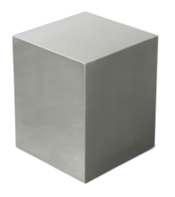To calculate density for a regular shaped solid:

1. Measure mass on balance
2. Calculate volume mathematically (length x width x height)
3. Calculate density = mass ÷ volume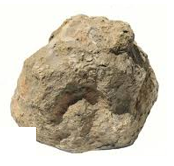To calculate density for an irregular solid:

1. Measure mass on balance
2. Measure volume using displacement – place the object in water and measure the amount of water displaced
3. Calculate density = mass ÷ volume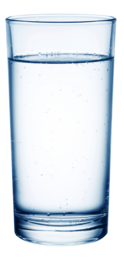To calculate density for a liquid:

1. Measure mass on balance (total mass – mass of container)
2. Measure volume using measuring cylinder
3. Calculate density = mass ÷ volume

Possible causes of error in these investigations include:

1. Inaccurate measurement of mass
2. Inaccurate measurement of length etc of regular shaped solid
3. Inaccurate measurement of displaced water

## Gas Pressure

### Brownian Motion

Individual molecules are too small to see directly but their motion can be observed by their effect on other particles. Particles in a gas move randomly. They move very fast. They collide with each other and the sides of the container. This is called Brownian motion.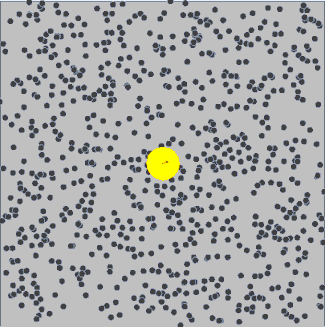Gas Pressure and Temperature

Increasing the temperature of a gas will increase the pressure as the particles move faster, causing more frequent collisions. This means that the pressure will increase (if the volume remains constant).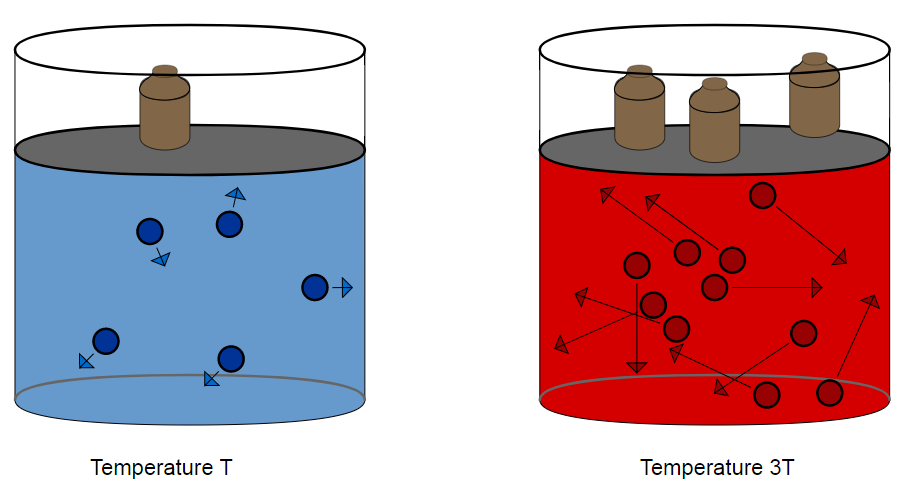Pressure and Volume

The same amount of air molecules in a smaller volume will exert more pressure as there will be more collisions: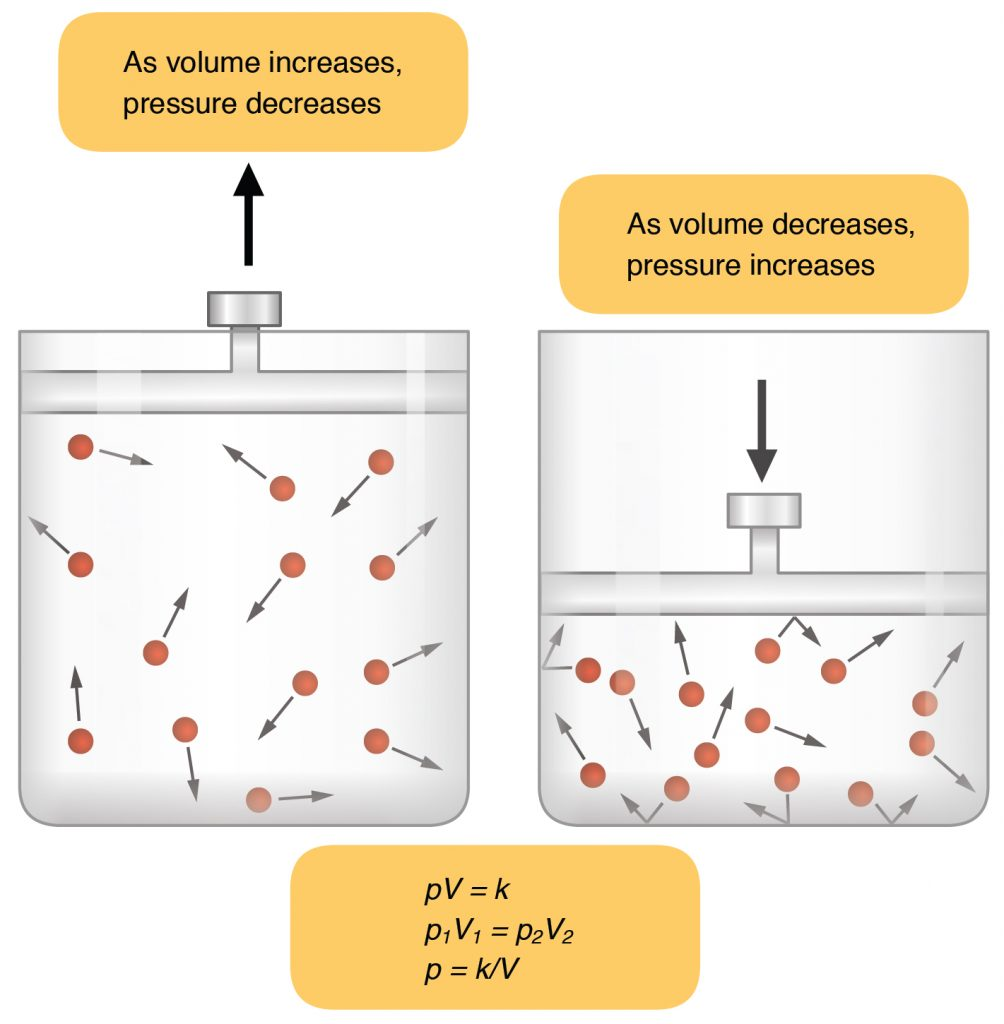## Boyle’s Law

Gas pressure is inversely proportional to volume

P x V = constant

P1V1 = P2V2

(you do NOT need to remember this equation for your exam)

What is the density of an object with mass 45kg and volume 5m3?
9
What is the mass of an object with volume 4m3 and density 5450kg/m3?
21800
What is the volume of an object with mass 420kg and density 140kg/m3?
3
A jeweller melts 0.5kg of gold to pour into a mould. Calculate how much energy was released as the gold solidified. (Latent heat of fusion of gold = 88000J/kg)
44000
A kettle fails to switch off and boils for 5 minutes, wasting 600 000 J of energy. Calculate the mass of water lost during this time. (Latent heat of vaporisation of water = 2,260,000J/kg)
0.265